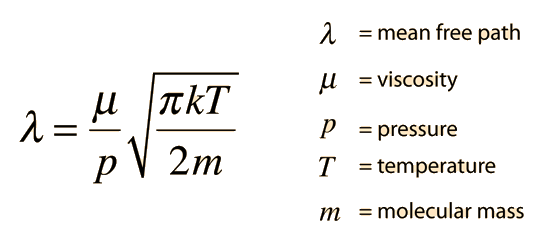# Mean Free Path Calculation for Hard Spheres and Viscous Gas

The mean free path of molecules in a gas can be modeled with the assumption that the molecules are hard spheres, and it can be modeled based on the viscosity of the gas. The intent here is to compare the results.

The mean free path equation depends upon the temperature and pressure as well as the molecular diameter.

For pressure P0 = mmHg =inHg =kPa

and temperature T=K = C =F =R ,

Molecules of diameter x 10-10 meters (angstroms)

 should have a mean free path of= x 10^m

which is times the molecular diameter

and times the average molecular separation of x 10^m.

The values for pressure, temperature, and molecular diameter may be changed above to recalculate the mean free path. A nominal molecular diameter of 0.3nm = 3 x 10-10 m will give you a reasonable approximation. The pressure required for a given mean free path can be calculated by changing the value of the mean free path λ above. The calculation is not designed to allow any other changes (their values will be replaced unchanged by the calculation.)

From the mean free path and the average velocity, the mean time between collisions and the frequency of collisions can be calculated.

Treating gas molecules as hard spheres is not always a good approximation. An alternate approach to mean free path is to force it to be consistent with measured values for the viscosity of the gas. The viscosity can be modeled by Sutherland's formula. The Since Sutherland's formula is an empirical fit of measured data, the following table of reference data is needed.

 Gas Sutherland'sconstant C T0°R μ0centiPoise massamu standard air 120 524.07 0.01827 28.966 ammonia, NH3 370 527.67 0.00982 17.02 carbon dioxide, CO2 240 527.67 0.01480 44.01 carbon monoxide, CO 118 518.67 0.01720 28.011 hydrogen, H2 72 528.93 0.00876 2.016 nitrogen, N2 111 540.99 0.01781 28.0134 oxygen, O2 127 526.05 0.02018 31.9988 sulfur dioxide, SO2 416 528.57 0.01254 64.06

For the temperature provided above a gas with
reference viscosity μ0 = centiPoise,

reference temperature T0 = Rankine,

and Sutherland's constant C =

should have a viscosity μ = centiPoise.

With the value of the viscosity, another approach to estimating the mean free path of a molecule in a gas is from the relationship:Then for gas molecules of mass m = amu,

the computed mean free path is λ = x 10^ m.

This calculation is just an investigation to see how closely the modeling of mean free path by hard sphere geometry and by projection from gas viscosity agree. For the projection from viscosity, standard air is used as the default, so that the values for air from the table above are substituted if no values are entered for the relevant parameters. Those values can be changed. As an example of model parameters, if you use 760mmHg for gas pressure, 0.3nm for molecular diameter, and 524.07R for temperature (the standard temperature for air in the table), the hard sphere calculation gives a mean free path of 99nm. If for the same temperature and using the standard value for air viscosity, 0.01827 centiPoise, the calculated mean free path is 65nm. If you adjust the hard sphere diameter to 0.3697nm, you bring the two estimates of mean free path into agreement, but I have no idea whether you can attach physical significance to this agreement. I would be interested in any physical data which might bear on this question.

References:

 Example of mean free path compared to molecular separation.
Index

Kinetic theory concepts

 HyperPhysics***** Thermodynamics R Nave
Go Back